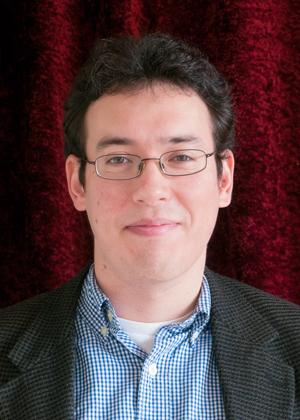Ryan Card, Ph.D.

Lecturer
Phone
253-692-4364
Campus Box
358436Degrees
Ph.D.
Mathematics
University of Washington Seattle
2009
M.S.
Mathematics
University of Washington Seattle
2006
B.S.
Mathematics
University of Washington Seattle
2002
B.S.
Statistics and Applied Computational Mathematical Science
University of Washington Seattle
2002
Introduction

I am a full-time lecturer teaching undergraduate mathematics courses. My expertise is in probability theory.

My research interests include stochastic processes, computational geometry, quantum computing and inverse problems.

Teaching
• TMATH 110 Intro to Statistics
• TMATH 120 Precalculus
• TMATH 124 Calculus I
• TMATH 125 Calculus II
• TMATH 126 Calculus III
• TMATH 214 Analytical Thinking
• TMATH 307 Intro to Diff Equations
• TMATH 390 Prob/Stats for Eng/Sci
• TESC 430 Environmental Modeling
CV or Resume
AttachmentSizeRyan Card CV80.57 KB